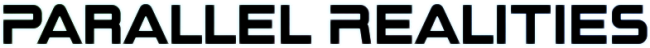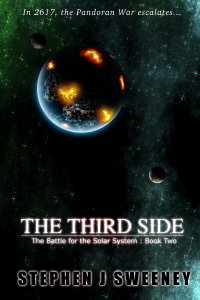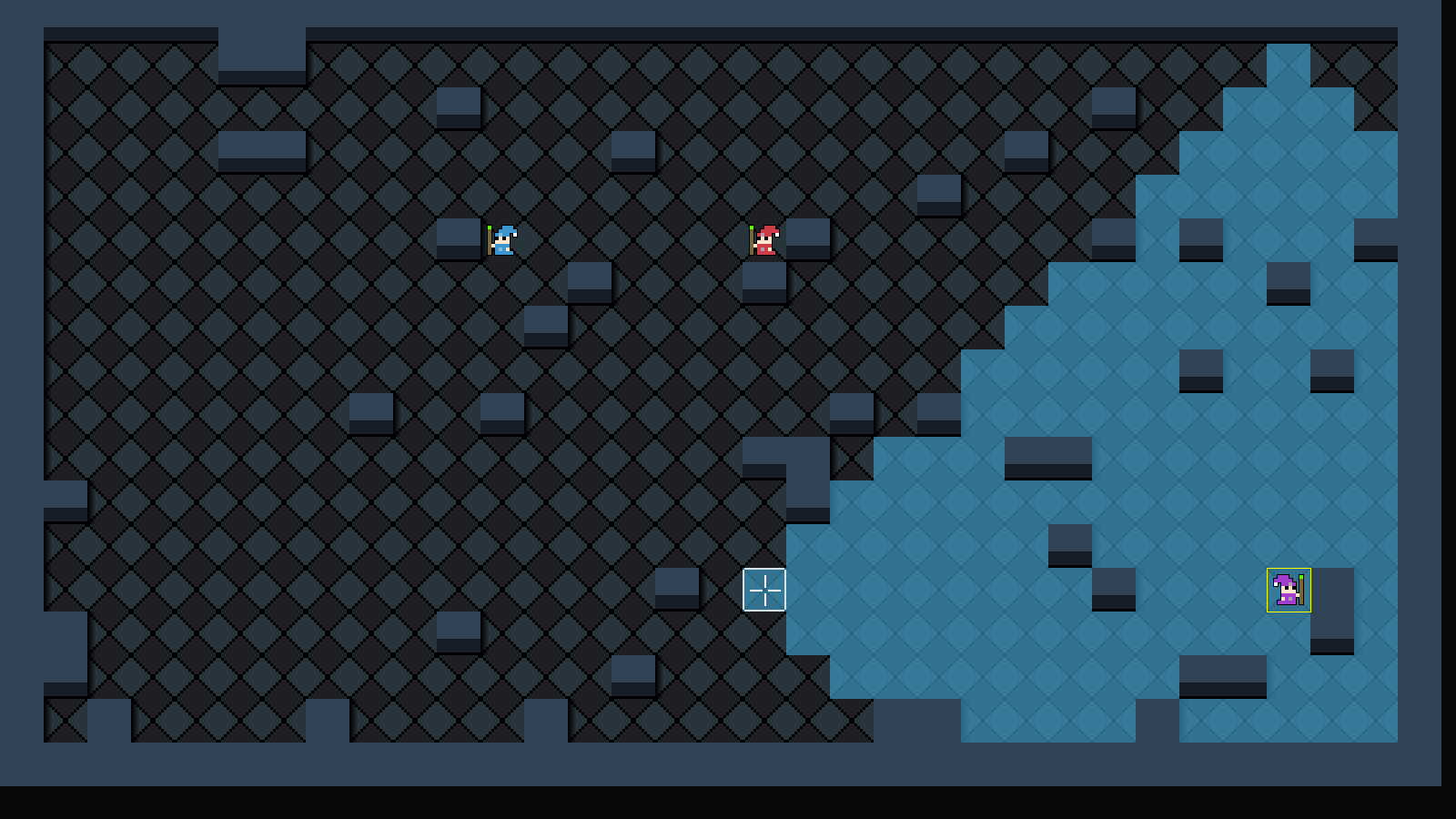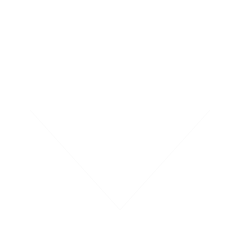Android Games

SDL2 turn-based strategy tutorial
Thu, 14th April 2022

Water Closet ported to PlayStation Vita
Tue, 4th January 2022

The Legend of Edgar 1.35
Sat, 1st January 2022

Achievements tutorial
Thu, 2nd December 2021

SDL2 Rogue tutorial
Thu, 30th September 2021

Tags

android (3)
battle-for-the-solar-system (9)
blob-wars (9)
brexit (1)
code (6)
edgar (7)
games (39)
lasagne-monsters (1)
making-of (5)
match3 (1)
numberblocksonline (1)
orb (2)
site (1)
tanx (4)
three-guys (3)
three-guys-apocalypse (3)
tutorials (10)
water-closet (4)

BooksThe Third Side (Battle for the Solar System, #2)

The White Knights have had their wings clipped. Shot down and stranded on a planet in independent space, the five pilots find themselves sitting directly in the path of the Pandoran war machine as it prepares to advance The Mission. But if they can somehow survive and find a way home, they might just discover something far more worrisome than that which destroyed an empire.

— A simple turn-based strategy game —
Part 3: Limiting movement

Note: this tutorial assumes knowledge of C, as well as prior tutorials.Introduction

Now that we have control over multiple unit, it's time to update our movement logic a bit. Right now, our units can move an unlimited range. It would be better if we restricted how far they can each move. In this part, we'll configure the movement range of each wizard separately, as well as display on screen the squares that the mage can move into.

Extract the archive, run cmake CMakeLists.txt, followed by make, and then use ./sdl2TBS03 to run the code. You will see a window open like the one above, showing three wizards in a small room, surrounded by walls. Clicking on a wizard more than once will toggle displaying the movement area. Tiles that can be moved into will be displayed in blue, while the other map squares will be dark. Click on a blue square to move into it. Notice that clicking on a dark square results in no movement. Select the wizards as per the previous tutorial. When you're finished, close the window to exit.

Inspecting the code

Displaying the movement limit for our wizards is very useful to the player, rather than having them attempt to guess how far they can move. There are quite a number of changes and updates requires to support this, so let's jump right in.

Starting with defs.h:

``````
enum {
SHOW_RANGE_NONE,
SHOW_RANGE_MOVE,
SHOW_RANGE_MAX
};
```
```

We've added an enum to handle displaying the movement range. SHOW_RANGE_NONE will mean don't display the movement range. SHOW_RANGE_MOVE will be used to instruct the game to display the movement range. SHOW_RANGE_MAX is used to assist with state looping, as we'll see later. Also, the reason we're using an enum here, rather than a simple flag is because in a later part we'll want to display the attack range, too.

``````
struct Entity {
unsigned int id;
int type;
char name[MAX_NAME_LENGTH];
int x;
int y;
int side;
int solid;
int facing;
AtlasImage *texture;
void (*data);
void (*draw) (Entity *self);
Entity *next;
};
```
```

The Entity struct now has a `data` field, as per past tutorials. This is to support extended data, such as the Unit struct:

``````
typedef struct {
int moveRange;
} Unit;
```
```

The Unit struct is new and will hold all the data about the unit. Right now, it has just one field: moveRange. Later, it can be expanded to hold data such as hit points, action points, etc.

The MapTile struct has also been tweaked:

``````
typedef struct {
int tile;
int inMoveRange;
} MapTile;
```
```

In addition to `tile`, we now have a field called inMoveRange. This is a flag that will state whether this MapTile falls within a unit's movement range.

Finally, we updated Stage:

``````
typedef struct {
unsigned int entityId;
MapTile map[MAP_WIDTH][MAP_HEIGHT];
Entity *currentEntity;
int animating;
int showRange;
struct {
int x;
int y;
} selectedTile;
} Stage;
```
```

Stage now includes a showRange field, that will make use of the SHOW_RANGE enums from defs. We'll see this in use in a little while.

Now, let's move over to units.c, where we've made several updates. Starting with initUnit:

``````
Unit *initUnit(Entity *e)
{
Unit *u;

u = malloc(sizeof(Unit));
memset(u, 0, sizeof(Unit));

e->data = u;
e->draw = draw;

return u;
}
```
```

We're now mallocing a Unit struct, memsetting it to zero all the data, and then assigning it to the entity's (`e`) `data` field. We're then returning the Unit, so that it can be tweaked further.

Next, we have a new function called updateUnitRanges:

``````
void updateUnitRanges(void)
{
int x, y;
Unit *u;

u = (Unit*) stage.currentEntity->data;

for (x = 0 ; x < MAP_WIDTH ; x++)
{
for (y = 0 ; y < MAP_HEIGHT ; y++)
{
stage.map[x][y].inMoveRange = 0;
}
}

testMoveRange(stage.currentEntity->x, stage.currentEntity->y, u->moveRange);
}
```
```

This function is very important to our movement range calculations and display. It will be called whenever we need to update the movement (and later, attack) ranges for the currently active entity. We start by extracting the Unit data from Stage's currentEntity, and then use two for-loops to reset all MapTiles' inMoveRange to 0. In effect, we'll be marking all the squares on our map as inaccessible. With that done, we're then calling testMoveRange, passing over the current entity's `x` and `y` fields, as well as the unit's moveRange. This is where we'll calculate movement range for the current unit.

testMoveRange itself follows:

``````
static void testMoveRange(int x, int y, int range)
{
Node *n;
int nx, ny;

{

for (nx = -1 ; nx <= 1 ; nx++)
{
for (ny = -1 ; ny <= 1 ; ny++)
{
if (nx != 0 || ny != 0)
{
addMoveRangeNode(n->x + nx, n->y + ny, x, y, range);
}
}
}

free(n);
}
}
```
```

What this function is doing is using a queue to test all the squares that can fall into the unit's movement range. It is important to note that we're not using for-loops here to simply mark the squares in a radius as being reachable, asecause they might not be. There could be walls in the way, and other objects such as solid entities could block routes. Therefore, we test one square at a time, adding its neighbours to the queue if it itself is accessible.

The function takes three parameters: `x`, `y`, and `range`. `x` and `y` are the starting position, while `range` is the maximum distance from that starting position we can move. We first prepare a linked list of Nodes (the same struct used in our A*) controlled by moveRangeHead and moveRangeTail. Next, we call addMoveRangeNode, passing through our `x` and `y` (twice, since this is the starting position), and our `range`. We'll explore addMoveRangeNode fully in a bit. For now, know that it will add to our linked list if a series of conditions are right.

We then start a while-loop, that will repeat until the linked list is empty (moveRangeHead's next is NULL). We grab the next Node in our linked list (assigning to `n`) and setup two for-loops, to add all the surrounding squares to our node queue, by calling addMoveRangeNode. Finally, we remove the current node from our queue.

So, quite simple: it's just a queue that will keep processing nodes and adding new ones until it is empty.

Now, let's look at addMoveRangeNode, where we are actually adding nodes to our queue:

``````
static void addMoveRangeNode(int x, int y, int ex, int ey, int range)
{
Node *n;

if (isInsideMap(x, y) && !stage.map[x][y].inMoveRange && abs(ex - x) + abs(ey - y) <= range && !isBlocked(x, y))
{
stage.map[x][y].inMoveRange = 1;

n = malloc(sizeof(Node));
memset(n, 0, sizeof(Node));
moveRangeTail->next = n;
moveRangeTail = n;

n->x = x;
n->y = y;
}
}
```
```

The function takes five arguments: `x` and `y`, the current node we're interested in. `ex` and `ey` represent the entity's starting position, while `range` is the maximum distance that a Node be.

In this function, we test to see if the position is valid to move into. To be a valid move point and a candidate for adding to our queue, the position must be inside our map, must not already be flagged as being inMoveRange, its distance from our starting location (`ex` and `ey`) must be less than or equal to `range`, and the location itself must not be otherwise blocked. With that determined, we set the relevant MapTile's inMoveRange to 1. We then malloc and memset a Node, add it to our queue, and set its `x` and `y` variables as the values of `x` and `y` that we passed into the function.

That's it! We can now determine the movement range of our unit. We can now look at how we integrate this system into the rest of our game.

First, let's turn to map.c, where we've updated drawMap:

``````
void drawMap(void)
{
int x, y, n;

for (x = 0 ; x < MAP_WIDTH ; x++)
{
for (y = 0 ; y < MAP_HEIGHT ; y++)
{
n = stage.map[x][y].tile;

blitAtlasImage(tiles[n], x * MAP_TILE_SIZE, y * MAP_TILE_SIZE, 0, SDL_FLIP_NONE);

if (!stage.animating && stage.showRange != SHOW_RANGE_NONE)
{
if (stage.map[x][y].inMoveRange)
{
blitAtlasImage(moveTile, x * MAP_TILE_SIZE, y * MAP_TILE_SIZE, 0, SDL_FLIP_NONE);
}
else
{
blitAtlasImage(darkTile, x * MAP_TILE_SIZE, y * MAP_TILE_SIZE, 0, SDL_FLIP_NONE);
}
}
}
}
}
```
```

As we can now determine the movement range, we are going to overlay this on our map tiles. After drawing the map tile, we're testing if Stage's `animation` flag is not set, and also whether we're displaying the movement range (Stage's showRange is not SHOW_RANGE_NONE). If so, we're then testing if the MapTile we're interested in has the inMoveRange flag set, and are calling blitAtlasImage, passing over an AtlasImage called moveTile. Otherwise, we're calling blitAtlasImage and passing over darkTile, causing the rest of the map to be dimmed.

``````
{
int i;
char filename[MAX_FILENAME_LENGTH];

for (i = 0 ; i < MAX_TILES ; i++)
{
sprintf(filename, "gfx/tiles/%d.png", i);

tiles[i] = getAtlasImage(filename, 0);
}

darkTile = getAtlasImage("gfx/tiles/dark.png", 1);

moveTile = getAtlasImage("gfx/tiles/move.png", 1);

routeTile = getAtlasImage("gfx/tiles/route.png", 1);
}
```
```

That's it for the updates to our map. We now head over to player.c, where we've tweaked doSelectUnit:

``````
static void doSelectUnit(void)
{
Entity *e;

if (app.mouse.buttons[SDL_BUTTON_LEFT])
{
e = getEntityAt(stage.selectedTile.x, stage.selectedTile.y);

if (e != NULL)
{
app.mouse.buttons[SDL_BUTTON_LEFT] = 0;

if (e == stage.currentEntity)
{
if (++stage.showRange >= SHOW_RANGE_MAX)
{
stage.showRange = SHOW_RANGE_NONE;
}
}
else
{
stage.currentEntity = e;

updateUnitRanges();
}
}
}

if (app.mouse.buttons[SDL_BUTTON_X1])
{
app.mouse.buttons[SDL_BUTTON_X1] = 0;

cyclePlayerUnits(-1);
}

if (app.mouse.buttons[SDL_BUTTON_X2])
{
app.mouse.buttons[SDL_BUTTON_X2] = 0;

cyclePlayerUnits(1);
}
}
```
```

Before, when evaluating the entity had been clicked on, we would swap to a new one if it wasn't NULL. Now, we are checking to see if the entity clicked on is the same as the current one. If so, we're incrementing Stage's showRange, and wrapping the value around if it is equal to or greater than SHOW_RANGE_MAX. In effect, this means that if we click on the same unit multiple times, we will cycle between displaying the movement range and showing just the map itself. If the entity is a different one, we will now swap to it, and call updateUnitRanges. This is important, as we want the allowed movement map tiles to be based on the unit we have now selected. Otherwise, we would retain the data from the previous unit.

doMoveUnit has also been updated:

``````
static void doMoveUnit(void)
{
MapTile *t;

if (app.mouse.buttons[SDL_BUTTON_LEFT])
{
app.mouse.buttons[SDL_BUTTON_LEFT] = 0;

t = &stage.map[stage.selectedTile.x][stage.selectedTile.y];

if (t->inMoveRange)
{
createAStarRoute(stage.currentEntity, stage.selectedTile.x, stage.selectedTile.y);
}
}
}
```
```

Previously, we would make a call to createAStarRoute regardless of where on the map the player clicked. Now, we're extracting the MapTile pointed to by Stage's selectedTile (assigned to a variable called `t`) and testing to see whether the inMoveRange flag is set. This means that the player units will now only move if the destination is within their move range.

addPlayerUnits has also seen a minor update:

``````
{
Entity *e;
int i, x, y;
char *names[] = {"Andy", "Danny", "Izzy"};

for (i = 0 ; i < NUM_PLAYER_UNITS ; i++)
{
e = initEntity(names[i]);

e->side = SIDE_PLAYER;

do
{
x = rand() % MAP_WIDTH;
y = rand() % MAP_HEIGHT;
}
while (isBlocked(x, y));

e->x = x;
e->y = y;

units[i] = e;
}

stage.currentEntity = units;

updateUnitRanges();
}
```
```

At the end of the function, we're calling updateUnitRanges. This is to ensure the ranges are set for the very first unit, when the game begins. If we didn't do this, the player would need to change units before they could control any of the mages.

We're almost done! We need just update mages.c, and stage.c. Let's look at mages.c first, starting with a tweak to initMage:

``````
Unit *initMage(Entity *e, char *name, char *filename)
{
Unit *u;

STRCPY(e->name, name);
e->type = ET_MAGE;
e->solid = 1;
e->texture = getAtlasImage(filename, 1);

u = initUnit(e);

return u;
}
```
```

As part of this function, we're now calling initUnit, passing over the entity (`e`) for which it is being created. We're then returning the created unit, so that it can be further modified by the calling function (without the need to extract it from the entity's `data` field).

We can see this in action in initAndyMage:

``````
void initAndyMage(Entity *e)
{
Unit *u;

u = initMage(e, "Andy", "gfx/units/andy.png");
u->moveRange = 10;
}
```
```

We're now assigning the result of initMage to a variable called `u`. We're then setting the unit's moveRange as 10. We have also updated initDannyMage with the same:

``````
void initDannyMage(Entity *e)
{
Unit *u;

u = initMage(e, "Danny", "gfx/units/danny.png");
u->moveRange = 9;
}
```
```

Danny, however, had a moveRange of 9, so he can't move quite as far. Finally, initIzzyMage has been adjusted:

``````
void initIzzyMage(Entity *e)
{
Unit *u;

u = initMage(e, "Izzy", "gfx/units/izzy.png");
u->moveRange = 12;
}
```
```

Izzy has a move range of 12, as she's a bit more nimble on her feet, compared to the boys.

That's it for mages.c. The last thing we need to update is the `logic` function in stage.c:

``````
static void logic(void)
{
int wasAnimating;

wasAnimating = stage.animating;

if (!stage.animating)
{
doPlayer();
}

doEntities();

doUnits();

doHud();

app.mouse.visible = !stage.animating;

if (wasAnimating && !stage.animating)
{
updateUnitRanges();
}
}
```
```

We've added in a new variable called wasAnimating, and assigning it the current value of Stage's animating flag. At the end of the function, we're testing to see if at the start of the function we were animating but are now not. In other words, our animation state has changed. If we're done animating, we're calling updateUnitRanges, as the currently active unit has finished moving or attack or taking another action, and so we can update our ranges once again. Since we know that actions result in various animation playing our, doing this check here allows us to centralize the logic.

Part 3 is done! We can now swap between our units, and have a system in place where we can limit how far they can move.

What needs to come next is action point handling, so we can limit how many actions a unit may take per turn. This will also incorporate some simple turn handling (as expected, since this is a turn-based strategy game, after all!).

Purchase

The source code for all parts of this tutorial (including assets) is available for purchase:

From itch.io

It is also available as part of the SDL2 tutorial bundle:

Directly

If you do not wish to create an itch.io account, you can also purchase the tutorial bundle using PayPal, and then download the tutorials directly from the main tutorials page.SDL2_Tutorials.tar.gz 56.76MB 23rd April 2022